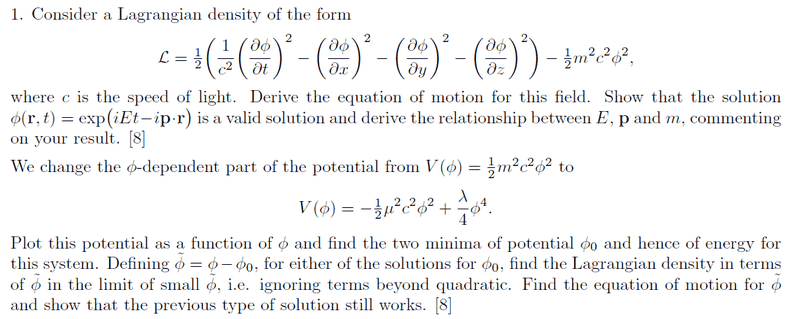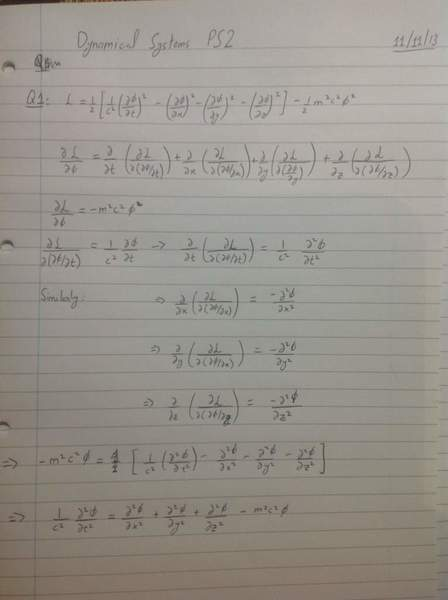# Derive Equation of motion using Lagrangian density?

Homework Statement [/b]The attempt at a solution[/b]I have done the first bit but don't know how to show that phi(r,t) is a solution to the equation of motion.

George Jones
Staff Emeritus
Gold Member
Substitute the given ##\phi## into the left side of your equation; substitute the given ##\phi## into the right side of your equation. After doing this, show that left = right.

Equivalently, but perhaps a little cleaner: take all your terms to the left side; show that substituting ##\phi## into the left side gives zero.

thanks, but how should I treat p.r term in the solution while differentiating with respect to (t,x,y,z)

George Jones
Staff Emeritus
$$\mathbf{p} \cdot \mathbf{r}=?$$